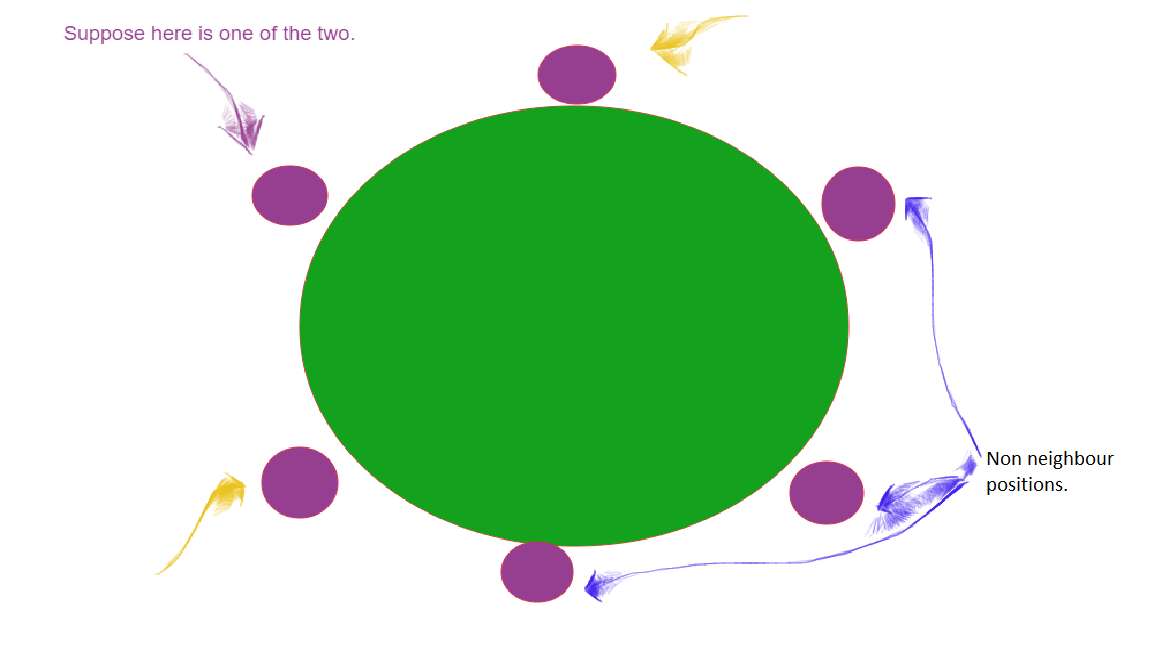Open in App
Not now

# Puzzle | Neighbors in a round table

• Difficulty Level : Easy
• Last Updated : 18 Jan, 2023

There are 6 persons seating on a round table in which two individual have the same names. What is the probability that the two same-named individuals will be neighbors?

Solution(Method 1): Total no of ways in which 6 persons can sit on a round table is (6-1)! = 5! = 120.
If we consider two same-named individuals as one person there are 5 persons who can sit in (5-1)! ways and these individuals can be seated together in 2! ways.

So, required probability =(2*(5-1)!)/(6-1)!= 2/5.
So, the answer is 2/5 = 0.4.

Solution(Method 2): We fix one of the same name guy in any position. Now we are left with 5 places out of which 2 can be seated neighbor.
Therefore, the answer is 2/5 = 0.4.My Personal Notes arrow_drop_up
Related Articles